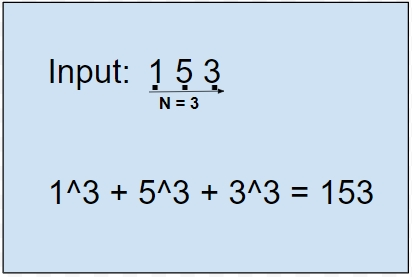# C Program for Armstrong Numbers

We are given a task where we have to check a number n entered by the user, whether it’s Armstrong or not.

An Armstrong number is when the sum of all the digits power by the number of digits or we can say order of digits n, is same as the digit.

So below is a simple representation how to find the Armstrong number −Formula −

wxyz…. = pow(w, n) +pow(x, n) + pow(y, n) + pow(z, n) + …..

## Algorithm

START
Step 1-> Declare a function to find the value after power operation on the number
int power(int a, int b)
Loop while b>0
Assign power =power * a
Decrement b by 1
End loop
Return power
End
Step 2-> Declare a function to count the order of a number
int count(int n)
Declare and set i as 0
Loop while n!=0
Increment i by 1
Divide n/10 and store back in n
End loop
Return i
End
Step 3-> Declare a function to check number is prime or not
int armstrong(int n)
Declare x and call function count(n) and assign the result to x
Declare rem = 0 and m=0 set with zero
Loop While n
Set rem = n %10
Set m = m + power(rem, x)
Set n as n/ 10
End Loop
Return m;
End
Step 4-> Declare main
int main(int argc, char const *argv[])
Declare and set n = 1634
Call function Armstrong and check if the value is equal
Print “it is armstrong number
End if
Else
Print number isn't an armstrong number
End
STOP

## Example

#include <stdio.h>
int power(int a, int b){
int power =1;
while(b>0){
power *= a;
b--;
}
return power;
}
int count(int n){
int i=0;
while(n!=0){
i++;
n = n/10;
}
return i;
}
int armstrong(int n){
int x = count(n);
int rem = 0, m=0;
while(n){
rem = n %10;
m += power(rem, x);
n /= 10;
}
return m;
}
int main(int argc, char const *argv[]){
int n = 1634;
if(n == armstrong(n)){
printf("%d is an armstrong number ",n);
}
else
printf("%d isn't an armstrong number ",n);
return 0;
}

## Output

1634 is an armstrong number